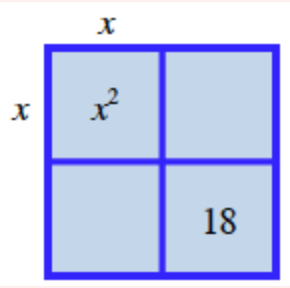### Home > A2C > Chapter 4 > Lesson 4.3.2 > Problem4-153

4-153.

If $x^2 + kx + 18$ is factorable, what are the possible values of $k$?${\begin{array} {c c c} \ \ \ 1&\ \ \ 2&\ \ \ 3&\ \ \ 6&\ \ \ 9&\ \ \ 18\\ -1&-2&-3&-6&-9&-18\\ \quad \ 1x&\quad \ 2x&\quad \ 3x&\quad \ 6x&\quad \ 9x&\quad \ 18x\\ \ -1x&\ -2x&\ -3x&\ -6x&\ -9x&\ -18x \end{array}}$

Find expressions that will complete the generic rectangle.

There are 6 answers: $+19$ and $-19$ are two of them.